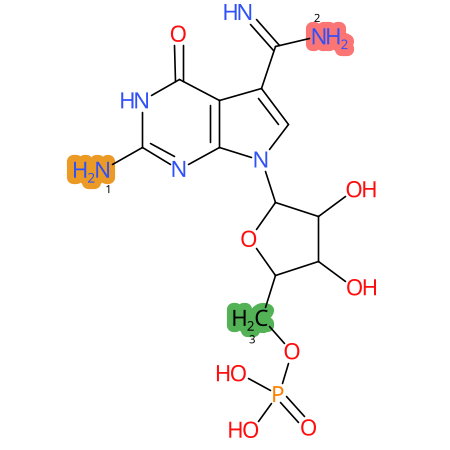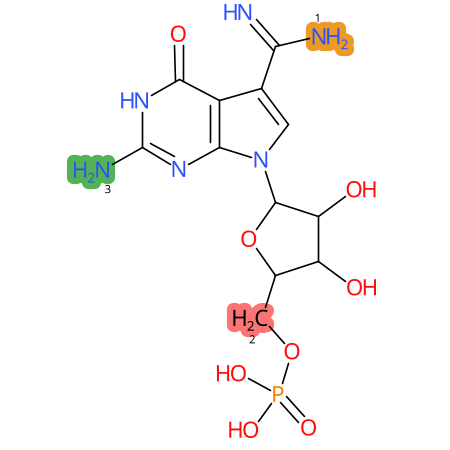Modomics - A Database of RNA Modifications

The molecule is shown in a ball-and-stick representation with the following colors for atoms :
Hydrogen (H): white Carbon (C): gray Oxygen (O): red Phosphorus (P): orange Nitrogen (N): blue Selenium (Se): gold Sulfur (S): yellow

### Summary

 Full name archaeosine-5'-monophosphate Short name pG+ MODOMICS code new 2000103551G MODOMICS code 103551G Nature of the modified residue Natural RNAMods code ( Residue unique ID 412 Related nucleosides 99

### Chemical information

 Sum formula C12H15N6O8P Type of moiety nucleotide SMILES N=C(N)c1c[n]([C@@H]2O[C@H](COP(=O)([O-])[O-])[C@@H](O)[C@H]2O)c2nc(N)[nH]c(=O)c12 logP -0.423 TPSA 258.49 Number of atoms 27 Number of Hydrogen Bond Acceptors 1 (HBA1) 10 Number of Hydrogen Bond Acceptors 2 (HBA2) 13 Number of Hydrogen Bond Donors (HBD) 6 InChI InChI=1S/C12H17N6O8P/c13-8(14)3-1-18(9-5(3)10(21)17-12(15)16-9)11-7(20)6(19)4(26-11)2-25-27(22,23)24/h1,4,6-7,11,19-20H,2H2,(H3,13,14)(H2,22,23,24)(H3,15,16,17,21)/p-2/t4-,6-,7-,11-/m1/s1 InChIKey ALFWBBQGYCDFHE-RPKMEZRRSA-L Search the molecule in external databases ChEMBL  ChemAgora  ChEBI  PubChem Compound Database  Ligand Expo  ChemSpider  WIPO

* Chemical properties calculated with Open Babel - O'Boyle et al. Open Babel: An open chemical toolbox. J Cheminform 3, 33 (2011) (link)

 2D .png .mol .mol2 .sdf .pdb .smi 3D .mol .mol2 .sdf .pdb

### Tautomers

 Tautomers SMILES N=C(N)c1cn(C2OC(COP(=O)([O-])[O-])C(O)C2O)c3[nH]c(=N)[nH]c(=O)c13 tautomer #0 N=C(N)c1cn(C2OC(COP(=O)([O-])[O-])C(O)C2O)c3nc(N)nc(O)c13 tautomer #1 N=C(N)c1cn(C2OC(COP(=O)([O-])[O-])C(O)C2O)c3[nH]c(N)nc(=O)c13 tautomer #2 N=C(N)c1cn(C2OC(COP(=O)([O-])[O-])C(O)C2O)c3nc(N)[nH]c(=O)c13 tautomer #3 N=C(N)c1cn(C2OC(COP(=O)([O-])[O-])C(O)C2O)c3nc(=N)[nH]c(O)c13 tautomer #4 N=C(N)C1=CN(C2OC(COP(=O)([O-])[O-])C(O)C2O)C3=NC(=N)NC(=O)C13 tautomer #5 N=C(N)C=1CN(C2OC(COP(=O)([O-])[O-])C(O)C2O)C3=NC(=N)NC(=O)C13 tautomer #6 N=C(N)c1cn(C2OC(COP(=O)([O-])[O-])C(O)C2O)c3nc(N)nc(O)c13 tautomer #7 N=C(N)C1=CN(C2OC(COP(=O)([O-])[O-])C(O)C2O)C3=NC(N)=NC(=O)C13 tautomer #8 N=C(N)C=1CN(C2OC(COP(=O)([O-])[O-])C(O)C2O)C3=NC(N)=NC(=O)C13 tautomer #9 N=C(N)c1cn(C2OC(COP(=O)([O-])[O-])C(O)C2O)c3[nH]c(=N)nc(O)c13 tautomer #10 N=C(N)C1=CN(C2OC(COP(=O)([O-])[O-])C(O)C2O)C3=NC(=N)N=C(O)C13 tautomer #11 N=C(N)C=1CN(C2OC(COP(=O)([O-])[O-])C(O)C2O)C3=NC(=N)N=C(O)C13 tautomer #12 Tautomer image Show Image

### Predicted CYP Metabolic Sites

CYP3A4 CYP2D6 CYP2C9* CYP Metabolic sites predicted with SMARTCyp. SMARTCyp is a method for prediction of which sites in a molecule that are most liable to metabolism by Cytochrome P450. It has been shown to be applicable to metabolism by the isoforms 1A2, 2A6, 2B6, 2C8, 2C19, 2E1, and 3A4 (CYP3A4), and specific models for the isoform 2C9 (CYP2C9) and isoform 2D6 (CYP2D6). CYP3A4, CYP2D6, and CYP2C9 are the three of the most important enzymes in drug metabolism since they are involved in the metabolism of more than half of the drugs used today. The three top-ranked atoms are highlighted. See: SmartCYP and SmartCYP - background; Patrik Rydberg, David E. Gloriam, Lars Olsen, The SMARTCyp cytochrome P450 metabolism prediction server, Bioinformatics, Volume 26, Issue 23, 1 December 2010, Pages 2988–2989 (link)

### LC-MS Information

 Monoisotopic mass None Average mass 402.257 [M+H]+ not available Product ions not available Normalized LC elution time * not available LC elution order/characteristics not available

* normalized to guanosine (G), measured with a RP C-18 column with acetonitrile/ammonium acetate as mobile phase.

Last modification of this entry: Sept. 22, 2023